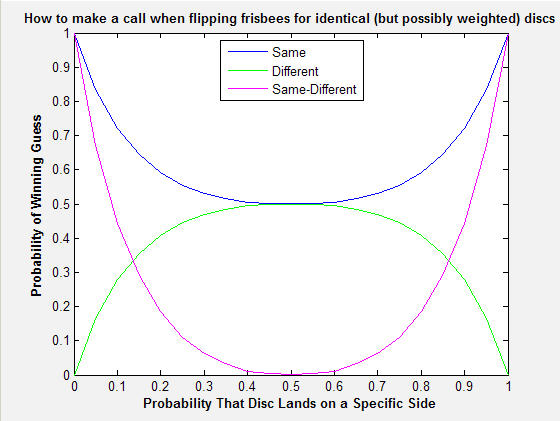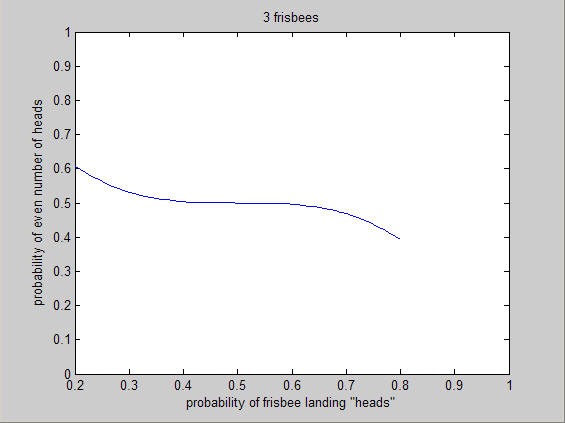# Puzzler: Ultimate Frisbee- call it! Wrap up

I do not know why I am still always amazed at the many different ways that simple problems are solved by different people. To end the suspense for everyone playing along at home,

“For two coins (i.e. Frisbees®) that have the same probability of being heads or tails (but not necessarily fair coins) you are at worst going to win 50% of the time when you choose ‘same’.” (Here is the original post)

My argument on the Ultimate Frisbee field was

“Imagine the two Frisbee come up heads 99% of the time, what do you choose?”

“Same!”

“Same”

“This logic holds all the way through, even to 50.00001. At 50% it just does not matter, so always choose same.”

The more rigorous and MATLAB proofs were more along these lines:This was a GUI that you watched as it went through a Monte Carlo simulation. Thanks Richard

Arman did a more traditional proof, citing Wikipedia

Let p be the probability of having tails.
The probability of having "different" is p(1-p)+(1-p)p.
The probability of having "same" is p^2+(1-p)^2.

From the arithmetic mean geometric mean inequality, we know that

p^2+(1-p)^2 >= 2*p(1-p) and equality holds if p=1-p which means p=1/2.

Therefore "same" is better choice for any p value.


There were many variations on this plot:I liked Zane’s here because it shows how much better off you are with ‘same’ for each value of unfairness in the coin.

Christopher won the challenge by going to the next level, pointing out that you could make these unfair Frisbees almost fair by flipping three and calling for an odd number (1 or 3) vs even number (0 or 2) of heads.Thank you everyone for playing, and finally putting this question to rest! Now the ethical question, knowing the coin flip is unfair, is it in the Spirit of The Game to let the other guy choose? Should we move to Christopher’s method of flipping three Frisbees?